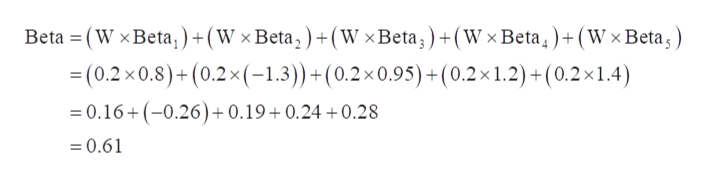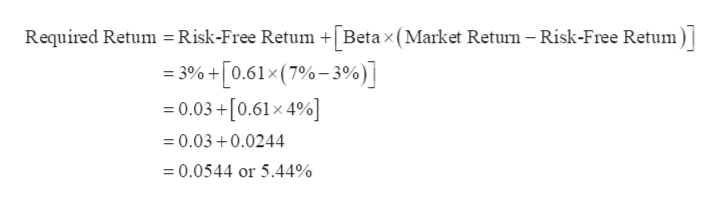# You invest in a portfolio of 5 stocks with an equal investment in each one. The betas of the 5 stocks are as follows: .8, -1.3, .95, 1.2 and 1.4. The risk-free return is 3% and the market return is 7%.A. Compute the beta of the portfolio.B. Compute the required return of the portfolio.

Question
14 views

You invest in a portfolio of 5 stocks with an equal investment in each one. The betas of the 5 stocks are as follows: .8, -1.3, .95, 1.2 and 1.4. The risk-free return is 3% and the market return is 7%.

A. Compute the beta of the portfolio.
B. Compute the required return of the portfolio.

check_circle

Step 1

A.

Number of Stocks = 5

Weight of Each Stock (W) = 1 ÷ 5 = 0.2

Beta1 = 0.8

Beta2 = -1.3

Beta3 = 0.95

Beta4 = 1.2

Beta5 = 1.4

Calculation of Beta of the Portfolio is as follows:help_outlineImage TranscriptioncloseBeta (W x Beta,) +(W x Beta,)+ (W x Beta)+(Wx Beta)+ (W x Beta) =(0.2 x0.8)+(0.2x(-1.3)+(0.2x 0.95) +(0.2 x 1.2) +(0.2x1.4) 0.16+(-0.26)+ 0.19+0.24+0.28 = 0.61 fullscreen
Step 2

B.

Beta = 0.61

Risk-Free Return = 3%

Market Return = 7%

Calculation of the req...help_outlineImage TranscriptioncloseRequired Retum =Risk-Free Retum Beta x (Market Return - Risk-Free Retum) 3%+[0.61x (7%-3%)] =0.03+[0.61x 4%] =0.030.0244 0.0544 or 5.44% fullscreen

### Want to see the full answer?

See Solution

#### Want to see this answer and more?

Solutions are written by subject experts who are available 24/7. Questions are typically answered within 1 hour.*

See Solution
*Response times may vary by subject and question.
Tagged in